# ACT Math Practice Test 2

Difficulty Level – 1: Easy

Directions: Solve each problem and then click on the correct answer. You are permitted to use a calculator on this test.

Congratulations - you have completed . You scored %%SCORE%% out of %%TOTAL%%. Your performance has been rated as %%RATING%%
 Question 1
The graph provided corresponds with which linear equation (assume this to be a line and not a line segment)?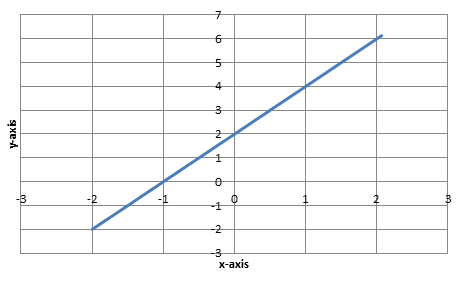A y = 3x + 2 B y = 2x + 2 C y = 2x D y = −2x − 2 E y = x + 2
Question 1 Explanation:
The correct answer is (B). Recall the slope-intercept form of a line:

y = mx + b where m is the slope and b is the y-intercept.

We can calculate the slope by finding the rise over the run from one point on the line to another. We can also use the formula: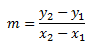It can be seen that the slope is equal to 2/1. We can also see that the y-intercept is at y = 2. Only answer choice (B) has a slope of 2 and a y-intercept of 2.
 Question 2
What is the value of 251.293 + 112.98 + 12.023 rounded to the nearest tenth?

 A 362 B 364.3 C 375.3 D 376 E 376.3
Question 2 Explanation:
The correct answer is (E). It is not always helpful to use a calculator to answer ACT questions; however, this question and those similar in style are perfect for calculator use. Perform the required operation with your calculator to find 376.296 and because we are rounding to the nearest tenth, and the hundredths place is a 9, we round up to 376.3.
 Question 3
If h(x) = 3x + 4, what is h(2x − 3)?

 A −6x + 5 B 6x − 5 C 3x + 1 D 5x − 2 E 6x
Question 3 Explanation:
The correct answer is (B). We solve this problem by replacing every x in h(x) with 2x − 3 and evaluating the expression:
h(2x − 3) = 3(2x − 3) + 4
= 6x − 9 + 4
= 6x − 5
 Question 4
In the figure below, what is the length of altitude y?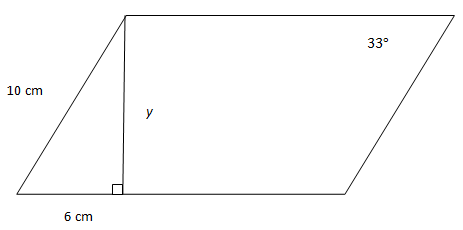A 7 cm B 8 cm C 9 cm D 10 cm E 12 cm
Question 4 Explanation:
The correct answer is (B). Sometimes questions will provide unnecessary information, in this case the angle measurement of the top right angle. We can focus exclusively on the right triangle shown and use Pythagorean Theorem, or the recognition of a Pythagorean triple to see that the length of y is 8 cm.
 Question 5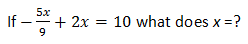A −13 BCD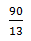E 13
Question 5 Explanation:
The correct answer is (D). Here we are trying to isolate the variable x using algebraic manipulation. Because we are provided an equation and not just an expression, we can begin by eliminating the denominator by multiplying the entire equation by 9:
−5x + 18x = 90
13x = 90
x = 90/13
 Question 6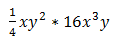What is this expression equivalent to?

 A 8x3y B 4x4y3 C 4x3y4 D 8x3y4 E 16¼x4y3
Question 6 Explanation:
The correct answer is (B). To simplify this expression we will first multiply ¼ by 16. And then using the rules of exponent multiplication:
x * x3 = x1 + 3 = x4
y2 * y = y2 + 1 = y3
Combining these values:
4x4y3
 Question 7
Each term in a sequence of numbers is generated by multiplying the preceding term by 3, and then adding 2. If the first term is −3, what will the 6th term be?

 A −487 B −165 C −57 D 57 E 165
Question 7 Explanation:
The correct answer is (A). Here is another question perfect for calculator use. Begin with –3 and follow the described procedure:
−3 * 3 + 2 = − 9 + 2 = −7
−7 * 3 + 2 = −21 + 2 = −19
−19 * 3 + 2 = −57 + 2 = −55
−55 * 3 + 2 = −165 + 2 = −163
−163 * 3 + 2 = −489 + 2 = −487
 Question 8This figure shows a line broken into 3 adjacent angles.

If 2y = 120, what is x + y = ?

 A 30 B 45 C 60 D 90 E 120
Question 8 Explanation:
The correct answer is (D). Begin by substituting the known value for 2y. Recall that a straight line is equal to 180°, so:
x + x + 2y = 180
2x + 120 = 180
2x = 60
x = 30
However, we are not asked to solve for x, we are asked for x + y. Because 2y = 120 → y = 60
x + y = 30 + 60 = 90
 Question 9
A bag of marbles contains 3 green marbles, 7 red marbles, 2 white marbles, and 8 black marbles. If a marble is randomly chosen, what is the probability that the marble will NOT be green or white?

 A 25% B 50% C 60% D 75% E 80%
Question 9 Explanation:
The correct answer is (D). Recall that the probability of an event happening is:In this case, we are looking for the probability of selecting a marble that is neither green nor white: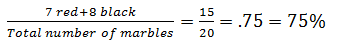Question 10
A family ordered 2 large pizzas for lunch. They ate 7/8 of one pizza and 1/4 of the other pizza. How much pizza was left for dinner?

 A 1/8 B 3/8 C 7/8 D 1 E 5/4
Question 10 Explanation:
The correct answer is (C). When dealing with addition and subtraction involving fractions we must remember to always convert everything to a common denominator. If the family consumed 7/8 of one and 1/4 or 2/8 of the other pizza, they consumed a total of 9/8 = 11/8. We can now subtract the 1/8 from the last remaining pizza to find 7/8.
Once you are finished, click the button below. Any items you have not completed will be marked incorrect.
There are 10 questions to complete.
 ← List →